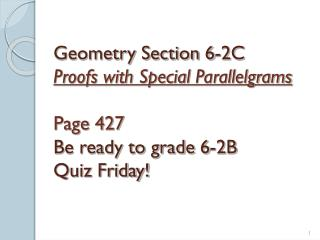DownloadDownload Presentation# Answers for 6-2B

Download Presentation## Answers for 6-2B

- - - - - - - - - - - - - - - - - - - - - - - - - - - E N D - - - - - - - - - - - - - - - - - - - - - - - - - - -
##### Presentation Transcript

1. Geometry Section 6-2C Proofs with Special ParallelgramsPage 427Be ready to grade 6-2BQuiz Friday!

2. Answers for 6-2B • No, consecutive angles are not supplementary (1 pt.) • Yes, opposite sides are congruent (1 pt.) • Yes, the diagonals bisect each other (1 pt.) • Yes, the diagonals bisect each other (1 pt.) • No, must have 2 sets of parallel sides (1 pt.) • No, must have 2 sets of congruent angles (1 pt.) • Yes, opposite angles are congruent (1 pt.) • Consecutive angles are supplementaryx = 24, y = 36 (3 pts.) • Alternate interior angles are congruentx = 10, y = 7 (3 pts.) • ÐQPS @ÐQRS and ÐPQR @ÐRSP (1 pt.) • QT = TS and PT = TR (or @ if segments) (1 pt.)

3. C B E Answers for 6-2B cont. A D • 10 pts. for the proof. (Order may be different, ask if you have a question.) • 1 pt. for a labeled drawing. 2 points for Given and Prove • AE @ CE Given • BE @ DE Given • ÐBEC @ÐAED Vertical angles are congruent • ÐAEB @ÐCED Vertical angles are congruent • DBEC @DAED SAS • DAEB @DCED SAS • ÐCBD @ÐBDA CPCTC • BC AD Alt. Int. angles theorem • ÐBCA @ÐCAD CPCTC • BA CD Alt. Int. angles theorem • ABCD is a parallel. Def. of parallelogram 28 pts. possible

4. GRADE SCALE – 28 POSSIBLE 27.5 – 98% 20.5 – 73% 27 – 96% 20 – 71% 26.5 – 95% 19.5 – 70% 26 – 93% 19 – 68% 25.5 – 91% 18.5 – 66% 25 – 89% 18 – 64% 24.5 – 88% 17.5 – 63% 24 – 86% 17 – 61% 23.5 – 84% 16.5 – 59% 23 – 82% 16 – 57% 22.5 – 80% 15.5 – 55% 22 – 79% 15 – 54% 21.5 – 77% 14.5 – 52% 21 – 75% 14 – 50% 4

5. Special Parallelograms: At the beginning of this chapter, we discussed the quadrilateral “Family Tree”. Since rhombuses, rectangles and squares are special parallelograms, their diagonals have some unique characteristics. We will use these characteristics to prove that a parallelogram is a rhombus, rectangle or square. Pg.427

6. Explore: (Smartboard program) Pg.428

7. Try It: Classify this quadrilateral if VX and YW bisect each other. Pg.429 Parallelogram

8. Try It: Classify this quadrilateral if VX and YW bisect each other and VX ^ YW. Pg.429 Rhombus

9. Try It: Classify this quadrilateral if VX and YW bisect each other and VX @ YW. Pg.429 Rectangle

10. Try It: Classify this quadrilateral if VX and YW bisect each other, VX ^ YW and VX @ YW. Pg.429 Square

11. Given: Parallelogram DEFG is a rectangle with diagonals DF and GE. Prove: DF @ GE DEFG is a rectangle Given ÐDGF andÐEFG are rt. Ð‘s Def. of rectangle DEFG is a parallelogram All rectangles are parallelograms DG@ EF Opp. sides of a parallelogram are @ Def. of right D DDGF andDEFG are rt. D‘s GF@ GF Reflexive property DDGF @DEFG LL Theorem DF@ GE CPCTC

12. Theorems: Theorems about diagonals of special parallelograms. A parallelogram is a… rhombus if and only if it’s diagonals are ^ . rectangle if and only if it’s diagonals are @ . Pg.429 square if and only if it’s diagonals are ^ and @ .

13. Exercises: Classify ABCD if AC and BD bisect each other. #1 Pg.430 Parallelogram THINK! What could each shape look like if the diagonals DON’T bisect each other? Don’t assume too much!

14. Exercises: Classify ABCD if AC and BD bisect each other and are congruent. #2 Pg.430 Rectangle

15. Exercises: Classify ABCD if AC and BD bisect each other and are perpendicular. #3 Pg.430 Rhombus

16. Exercises: Classify ABCD if AC and BD bisect each other are perpendicular and congruent. #4 Pg.430 Square

17. Exercises: Find the values of x and y for the quadrilateral. Justify your answer. #13 Pg.431 For a rectangle, diagonals must bisect and be congruent. y - 5 = 15 3x = 15 x = 5 y = 20

18. Exercises: Find the values of x and y for the quadrilateral. Justify your answer. For a rectangle, diagonals must bisect and be congruent; and opposite sides are congruent. #14 Pg.431 The corners of a rectangle are right angles. Therefore… Therefore, using the SSS theorem, the triangles are congruent and CPCTC says… x + y = 90 x = 25 25 + y = 90 y = 65

19. Exercises: Find the value of x for the quadrilateral. Justify your answer. For a square, the diagonals will bisect each angle and each corner will = 90o. #15 Pg.431 Therefore, 2x – 6 + 2x – 6 = 90 4x = 90 + 12 4x = 102 x = 25.5

20. Homework: Practice 6-2C Quiz Friday